\$referrer_host = www.google.com. Significant Figures Practice Problems - Chemistry Steps

## General Chemistry

In the previous two posts, we discussed the meaning of significant figures, counting them in a number, and the rules of using them in addition, subtraction, multiplication, and division. These are the main ideas you need to remember when working on problems involving significant figures. When counting them, remember that significant figures apply to measured numbers such as length, volume speed and etc. because of the uncertainty in those measurements. Any standard number given by a definition is considered to have an infinite number of significant figures.

Now, when identifying them in a number, remember that all nonzero digits are significant. Whether a zero is significant or not, is determined based on its position.  Read the article about significant figures for more information.

For the mathematical operations, you need to remember that in multiplication and subtraction, the answer is rounded off the same number of significant figures as the initial one with the fewest.

For addition and subtraction, you need to go based on the number of decimal places. In any calculation though, you want to keep in mind that the idea is to drop any numbers that are not significant. Read the article on significant figures in calculations for more details.

Check Also

#### Practice

1.

How many significant figures are in each number?

a) 632

b) 45.0

c) 4706.0

d) 0.0075

e) 0.006840

f) 0.15000

g) 2.30 x 104

h) 5,000

i) 1.234 x 10-3

j) 340.010

k) 0.004050

a)

3 S.F.

b)

3 S.F

c)

5 S.F.

d)

2 S.F.

e)

4 S.F.

f)

5 S.F.

g)

3 S.F.

h)

1 S.F.

i)

4 S.F.

Solution

a) 632

All the digits are nonzero, therefore, there are 3 S.F.

b) 45.0

The zero comes after a decimal place, therefore it is 3 S.F. and overall, there are 3 S.F.

c) 4706.0

One of the zeros appears between two nonzero digits, and the other comes after a decimal place, therefore they are both significant which makes 5 S.F. in total.

d) 0.0075

The leading zeros are not significant, therefore we have 2 S.F.

e) 0.006840

The leading zeros are not significant, while the trailing zero is significant therefore we have 4 S.F.

f) 0.15000

The leading zeros are not significant, while the trailing zeros are significant therefore, we have 5 S.F.

g) 2.30 x 104

We look at the number before the exponent, and here it has 3 S.F.

h) 5,000

Only 1 S.F. because there is no decimal, and the trailing zeros are not significant.

i) 1.234 x 10-3

We look at the number before the exponent, and here it has 4 S.F.

j) 340.010

All the zeroes are significant, so we have 6 S.F.

k) 0.004050

The leading zeros are not significant, while the trailing zero and the one in the middle are significant therefore, we have 4 S.F.

2.

Round off the following numbers as indicated.

a) 125.64 to 3 significant figures

b) 26547 to 4 significant figures in scientific notation

c) 9849 to 2 significant figures in scientific notation

d) 748965 to 5 significant figures in scientific notation

a)

126

b)

2.655 x 104

c)

9.8 x 103

d)

7.4897 x 105

Solution

a) 125.64 to 3 significant figures

We keep the first three numbers and round up the 5 to 6: 126

b) 26547 to 4 significant figures in scientific notation

Keep four numbers by rounding up the 4 to 5 and add the corresponding exponent. Remember, to determine the exponent power based on the initial number before dropping the extra digits: 2.655 x 104

c) 9849 to 2 significant figures in scientific notation

Keep two numbers and add the corresponding exponent: 9.8 x 103

d) 748965 to 5 significant figures in scientific notation

Keep five numbers by rounding up the 6 to 7 and add the corresponding exponent. Remember, to determine the exponent power based on the initial number before dropping the extra digits: 7.4897 x 105

3.

Which numbers are exact indicating they have an unlimited number of significant figures?

a) MPG of the car showing as 28 miles per gallon

b) 1 km = 1000 m

c) A bag of potatoes labeled as 3 lb

d) A class with 26 students

e) 1 ft = 12 in

a)

Not exact

b)

Exact

c)

Not exact

d)

Exact

e)

Exact

Solution

a) MPG of the car showing as 28 miles per gallon

This is a measured number and therefore, it is not an exact number.

b) 1 km = 1000 m

This is a definition and therefore the numbers are exact.

c) A bag of potatoes labeled as 3 lb

The potatoes were weighed using a scale so it is a measurement and the number is not exact.

d) A class with 26 students

This is an exact number as we do not measure it.

e) 1 ft = 12 in

This is a definition and therefore the numbers are exact.

4.

Perform the following calculations and report the answers in the correct number of significant figures.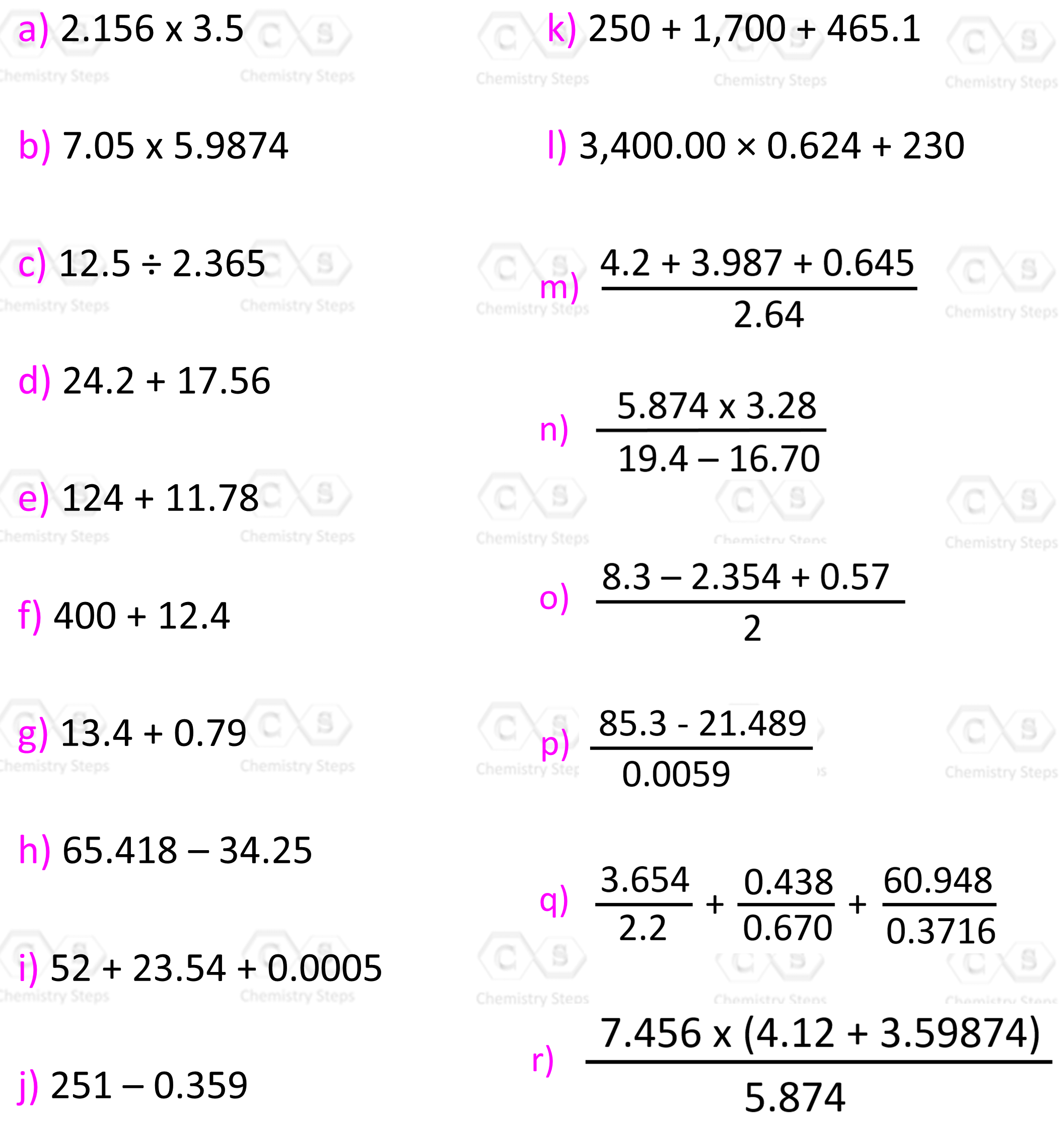a)

7.5

b)

42.2

c)

5.29

d)

41.8

e)

136

f)

400

g)

14.2

h)

31.17

i)

76

j)

251

k)

2,400

l)

2,400

m)

3.3

n)

7.1

o)

3

p)

11,000

q)

166.3

r)

9.80

Solution

a) 2.156 x 3.5

2.156 x 3.5 = 7.546 ≈ 7.5

In multiplication and division, the answer is rounded to the number with the least number of significant figures which is 3.5 here. Therefore, the answer should have two significant figures.

b) 7.05 x 5.9874

7.05 x 5.9874 = 42.2117 ≈ 42.2

In multiplication and division, the answer is rounded to the number with the least number of significant figures which is 7.05 here. Therefore, the answer should have three significant figures.

c) 12.5 ÷ 2.365

12.5 ÷ 2.365 = 5.2854122 ≈ 5.29

In multiplication and division, the answer is rounded to the number with the least number of significant figures which is 12.5 here. Therefore, the answer should have three significant figures.

d) 24.2 + 17.56

24.2 + 17.56 = 41.76 ≈ 41.8

In addition and subtraction, the answer should have as many decimal places as the number with the fewest. 24.2 has one decimal place and therefore, the answer should also be rounded off to one decimal place.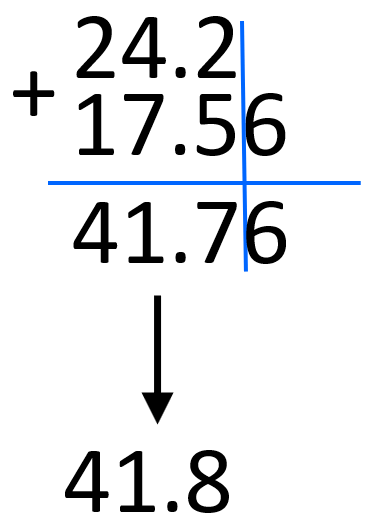e) 124 + 11.78

124 + 11.78 = 135.78 ≈ 136

In addition and subtraction, the answer should have as many decimal places as the number with the fewest. 124 has no decimal places and the least certain digit is in ones place, therefore, the answer should also be rounded off to the ones place.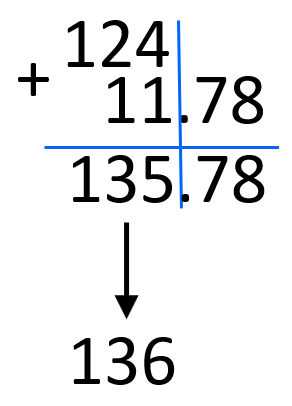f) 400 + 12.4

400 + 12.4 = 412.4 ≈ 400

In addition and subtraction, the answer should have as many decimal places as the number with the fewest. 400 has no decimal places and the least certain digit is in hundreds place, therefore, the answer should also be rounded off to the hundreds place.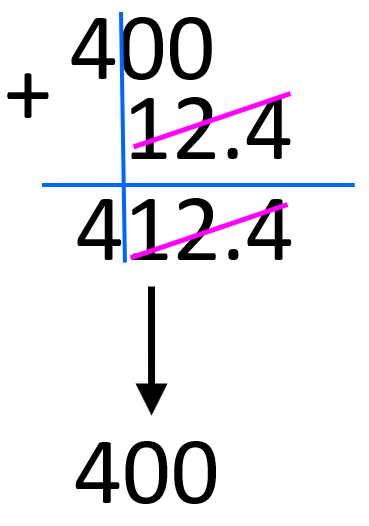g) 13.4 + 0.79

13.4 + 0.79 = 14.19 ≈ 14.2

In addition and subtraction, the answer should have as many decimal places as the number with the fewest. 13.4 has one decimal place and therefore, the answer should also be rounded off to one decimal place.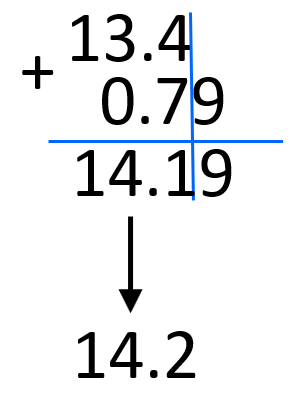h) 65.418 – 34.25

65.418 – 34.25 = 31.168 ≈ 31.17

In addition and subtraction, the answer should have as many decimal places as the number with the fewest. 34.25 has two decimal places and therefore, the answer should also be rounded off to two decimal places.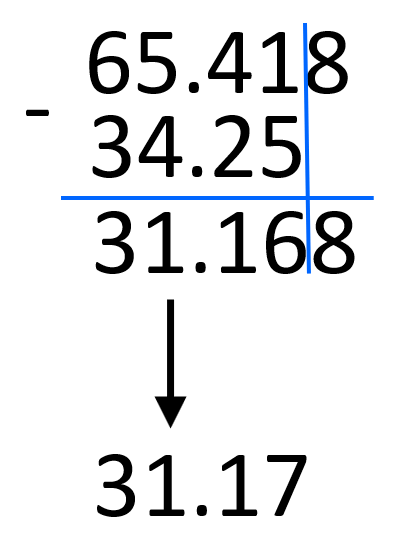i) 52 + 23.54 + 0.0005

52 + 23.54 + 0.0005 = 75.5405 ≈ 76

In addition and subtraction, the answer should have as many decimal places as the number with the fewest. 52 has no decimal places and the least certain digit is in ones place, therefore, the answer should also be rounded off to the ones place.j) 251 – 0.359 = 250.641 ≈ 251

In addition and subtraction, the answer should have as many decimal places as the number with the fewest. 251 has no decimal places and the least certain digit is in ones place, therefore, the answer should also be rounded off to the ones place.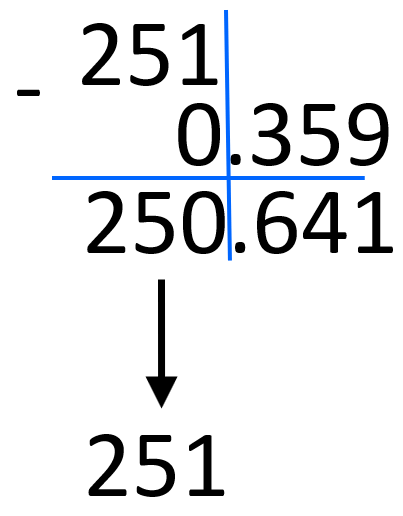k) 250 + 1,700 + 465.1 = 2,415.1 ≈ 2,400

In addition and subtraction, the answer should have as many decimal places as the number with the fewest. 1,700 has no decimal places and the least certain digit is in hundreds place, therefore, the answer should also be rounded off to the hundreds place.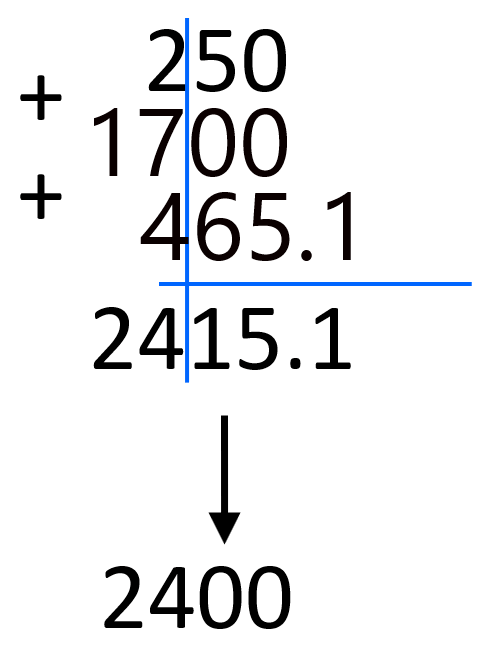l) 3,400.00 × 0.624 + 230 = 2,121.6 + 230 = 2,351.6 ≈ 2,400

According to the order of mathematical operations, the first step is the multiplication which gives 2,121.6. Now, this number should only have 2 significant figures but instead of rounding it we keep track of two significant figures and do the addition. The addition gives 2,351.6 and we should round this to the hundreds place because that was the certainty of 3,400.

You can  also do this by drawing the line where the significant figures must end, and this line is at the hundreds place: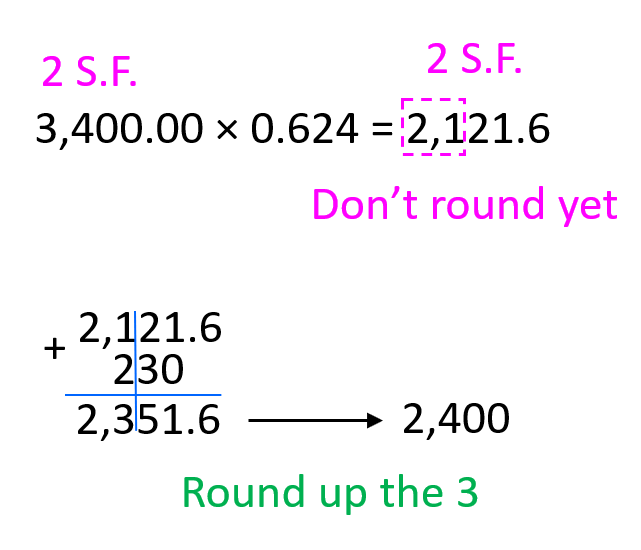m) First, we need to do the addition in the numerator and keep track of the significant figures based on the least number of decimal places. In this case, one decimal place indicates 2 significant figures which is what we use for the next step.n) Based on 3.28, the product in the numerator should have 3 significant figures. In the denominator, the result should have one decimal place which projects into 2 significant figures, and therefore, the final answer should also have two significant figures.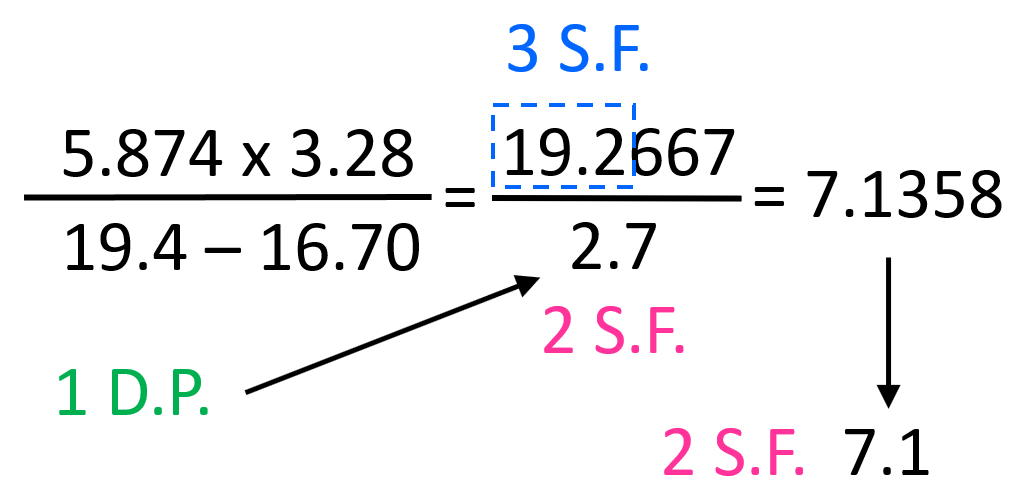o) First, we need to do the subtraction and addition in the numerator and keep track of the significant figures based on the least number of decimal places. In this case, one decimal place indicates 2 significant figures. However, the number in the denominator has only one significant figure and therefore, the answer is rounded to 1 significant figure.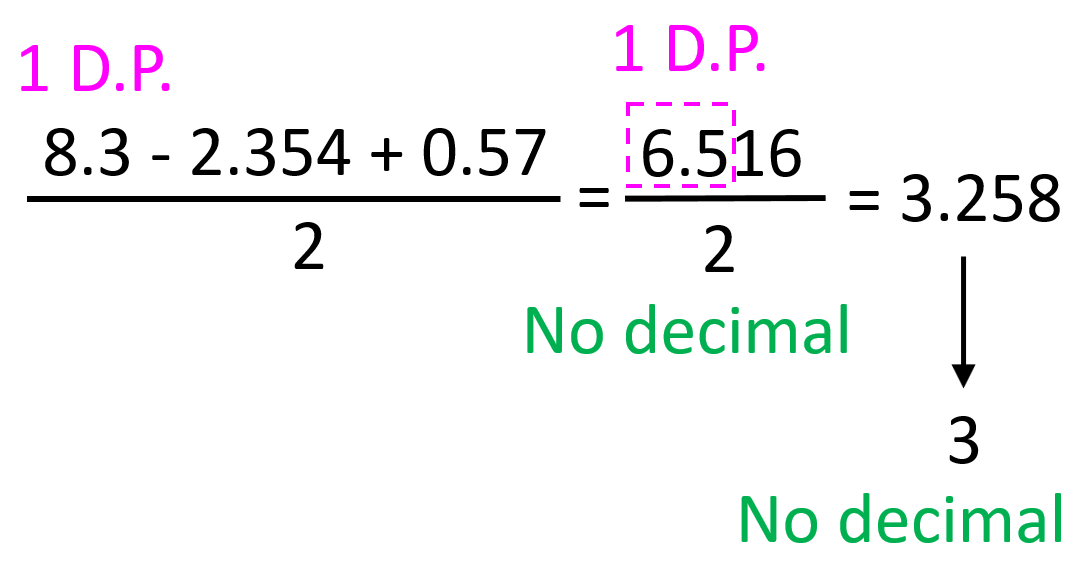p) First, we need to do the subtraction in the numerator and keep track of the significant figures based on the least number of decimal places. In this case, one decimal place projects into 3 significant figures. However, the number in the denominator has only two significant figures which is how the final answer is rounded off.q) First do the division of each fraction and keep track of the significant figures by rounding them yet. Identify the answer with the least number of decimal places to use for the answer after the additions. Because of 2.2, the result of the first fraction, 1.6609 should be used as if it has only one decimal place which dictates the certainty of the final answer.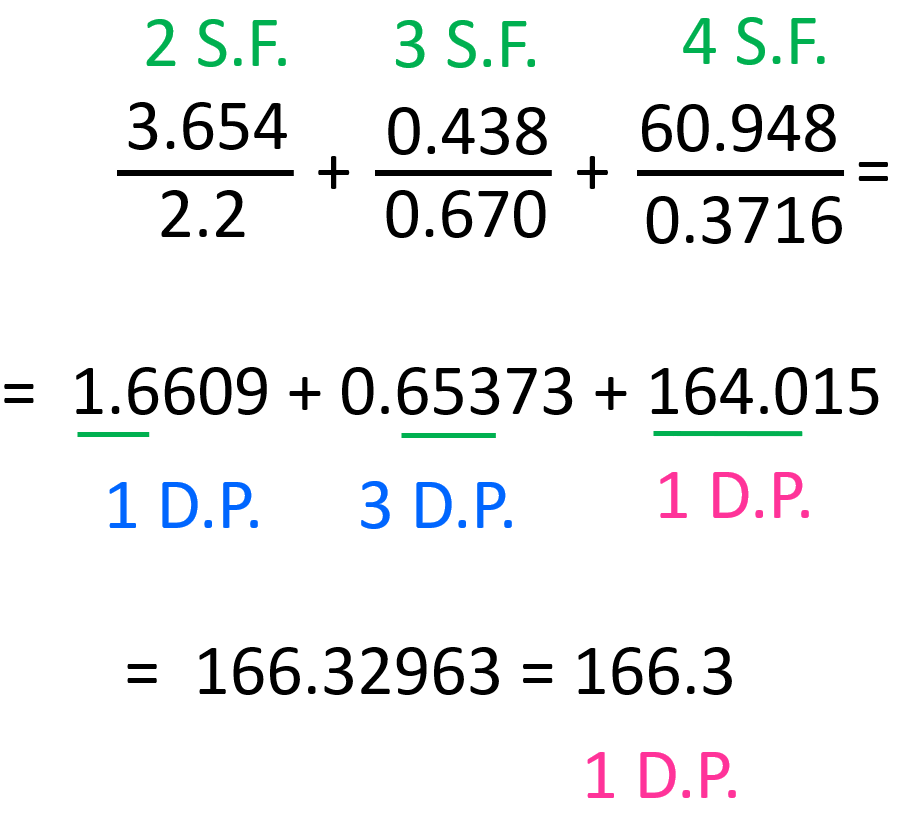r) First, we do the addition in the parenthesis and keep track of the number of significant figures based on the decimal places. 2 decimal place projects, not 3 significant figures which is less certain than 7.456 and 5.874, and therefore the answer must also have 3 significant figures.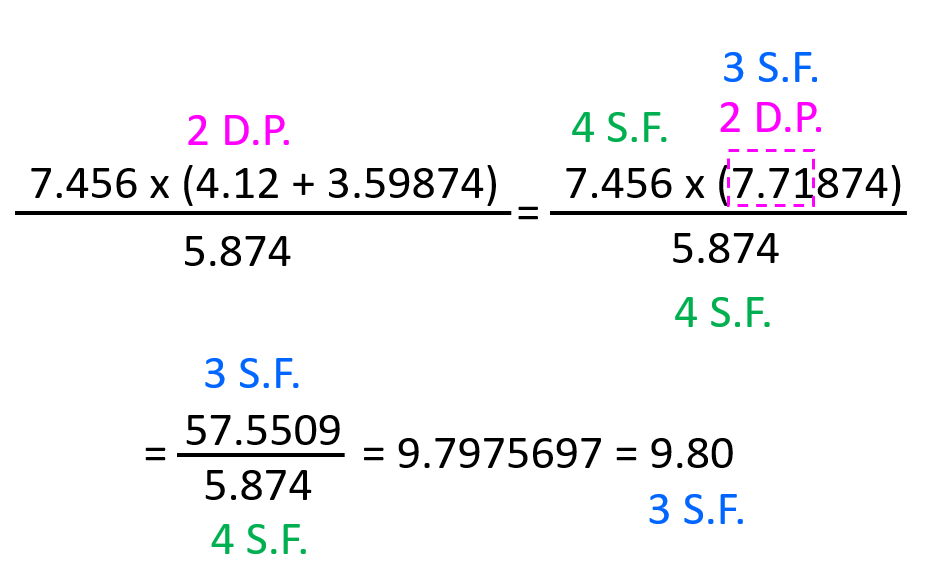1685274684